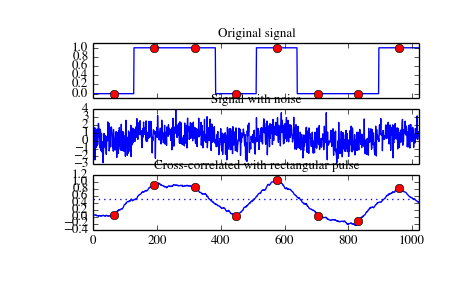# scipy.signal.correlate¶

scipy.signal.correlate(in1, in2, mode='full')[source]

Cross-correlate two N-dimensional arrays.

Cross-correlate in1 and in2, with the output size determined by the mode argument.

Parameters: in1 : array_like First input. in2 : array_like Second input. Should have the same number of dimensions as in1; if sizes of in1 and in2 are not equal then in1 has to be the larger array. mode : str {‘full’, ‘valid’, ‘same’}, optional A string indicating the size of the output: full The output is the full discrete linear cross-correlation of the inputs. (Default) valid The output consists only of those elements that do not rely on the zero-padding. same The output is the same size as in1, centered with respect to the ‘full’ output. correlate : array An N-dimensional array containing a subset of the discrete linear cross-correlation of in1 with in2.

Notes

The correlation z of two d-dimensional arrays x and y is defined as:

z[...,k,...] = sum[..., i_l, ...]
x[..., i_l,...] * conj(y[..., i_l + k,...])

Examples

Implement a matched filter using cross-correlation, to recover a signal that has passed through a noisy channel.

>>> from scipy import signal
>>> sig = np.repeat([0., 1., 1., 0., 1., 0., 0., 1.], 128)
>>> sig_noise = sig + np.random.randn(len(sig))
>>> corr = signal.correlate(sig_noise, np.ones(128), mode='same') / 128

>>> import matplotlib.pyplot as plt
>>> clock = np.arange(64, len(sig), 128)
>>> fig, (ax_orig, ax_noise, ax_corr) = plt.subplots(3, 1, sharex=True)
>>> ax_orig.plot(sig)
>>> ax_orig.plot(clock, sig[clock], 'ro')
>>> ax_orig.set_title('Original signal')
>>> ax_noise.plot(sig_noise)
>>> ax_noise.set_title('Signal with noise')
>>> ax_corr.plot(corr)
>>> ax_corr.plot(clock, corr[clock], 'ro')
>>> ax_corr.axhline(0.5, ls=':')
>>> ax_corr.set_title('Cross-correlated with rectangular pulse')
>>> ax_orig.margins(0, 0.1)
>>> fig.show()#### Previous topic

scipy.signal.convolve

#### Next topic

scipy.signal.fftconvolve